﻿ 基于多状态驾驶环境的安全驾驶可靠度评估 Reliability Assessment of Safe Driving Based on Multi-State Driving Environment

Operations Research and Fuzziology
Vol.08 No.03(2018), Article ID:26013,7 pages
10.12677/ORF.2018.83011

Reliability Assessment of Safe Driving Based on Multi-State Driving Environment

Kun Shi, Yongjin Zhang, Chaoyong Sun

School of Mathematics and Physics, Anhui University of Technology, Ma’anshan AnhuiReceived: Jul. 2nd, 2018; accepted: Jul. 12th, 2018; published: Jul. 20th, 2018ABSTRACT

The driving environment directly affects the perception, judgement and operation of vehicle drivers. First, through the analysis of the influencing factors of safety driving factors, the influence factors are divided into six aspects. Then, the weight of each factor is calculated by the analytic hierarchy process (AHP), and the factors are divided into multiple states and the factor state table is formulated. The membership degree of the measured values is allocated by the fuzzy mathematics membership function, the fuzzy matrix of membership degree is constructed, and the safety driving reliability is calculated based on the factor weight and the fuzzy matrix method. Finally, taking the safe driving in sunny weather in the daytime as an example, the analysis shows that the fuzzy analytic hierarchy process (AHP) is effective in evaluating the safety driving reliability.

Keywords:Multi State Environment, Analytic Hierarchy Process, Membership Function, Fuzzy Matrix, ReliabilityCopyright © 2018 by authors and Hans Publishers Inc.1. 引言

2. 评价因子与权重确定

2.1. 评价因子

1) 道路交通法规

2) 路况

3) 车速

4) 温度

5) 照明

6) 可视距离

2.2. 因子权重确定

1) 建立递阶层次结构

2) 构造判断矩阵并赋值

3) 计算因子权重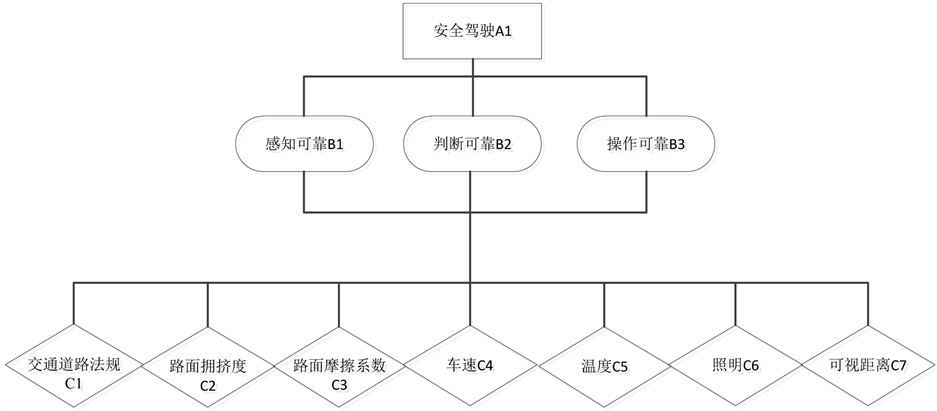Figure 1. Hierarchical structure diagram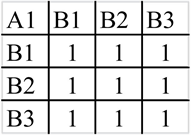Figure 2. Judgment matrix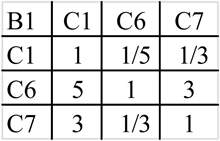Figure 3. Judgment matrix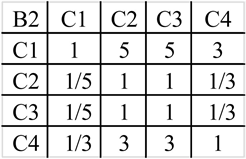Figure 4. Judgment matrix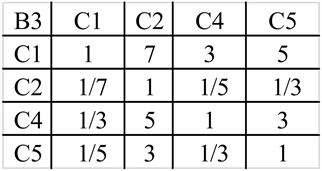Figure 5. Judgment matrix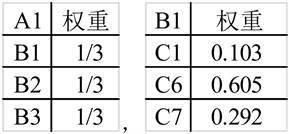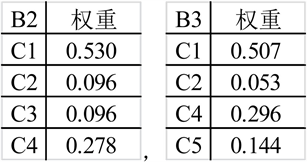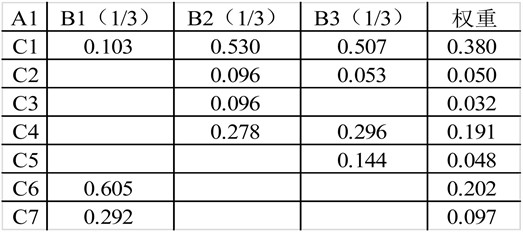$A=\left(\begin{array}{ccccccc}0.38& 0.05& 0.032& 0.191& 0.048& 0.202& 0.097\end{array}\right)$

4) 因子状态分类表的制定

3. 隶属函数确定与计算可靠度

3.1. 隶属函数

${Z}_{1}\left(x\right)=\left\{\begin{array}{l}1\text{ }\text{ }\text{ }\text{\hspace{0.17em}}\text{\hspace{0.17em}}\text{\hspace{0.17em}}\text{\hspace{0.17em}}\text{\hspace{0.17em}}\text{\hspace{0.17em}}\text{\hspace{0.17em}}\text{\hspace{0.17em}}\text{\hspace{0.17em}}\text{\hspace{0.17em}}\text{\hspace{0.17em}}\text{\hspace{0.17em}}\text{\hspace{0.17em}}\text{\hspace{0.17em}}\text{\hspace{0.17em}}\text{\hspace{0.17em}}x<{u}_{1}\\ \frac{{u}_{2}-x}{{u}_{2}-{u}_{1}}\text{\hspace{0.17em}}\text{\hspace{0.17em}}\text{\hspace{0.17em}}\text{\hspace{0.17em}}\text{\hspace{0.17em}}{u}_{1}\le x\le {u}_{2}\\ 0\text{ }\text{ }\text{ }\text{ }\text{ }\text{ }\text{ }\text{ }\text{ }\text{ }\text{ }\text{ }\text{ }\text{ }\text{ }\text{ }\text{ }\text{ }\text{ }\text{\hspace{0.17em}}\text{\hspace{0.17em}}\text{\hspace{0.17em}}\text{\hspace{0.17em}}\text{\hspace{0.17em}}\text{\hspace{0.17em}}\text{\hspace{0.17em}}\text{\hspace{0.17em}}\text{\hspace{0.17em}}x>{u}_{2}\end{array}$

${Z}_{3}\left(x\right)=\left\{\begin{array}{l}0\text{ }\text{ }\text{ }\text{ }\text{ }\text{ }\text{ }\text{ }\text{ }\text{ }\text{ }\text{ }\text{ }\text{ }\text{\hspace{0.17em}}\text{\hspace{0.17em}}\text{\hspace{0.17em}}\text{\hspace{0.17em}}\text{\hspace{0.17em}}\text{\hspace{0.17em}}x<{u}_{2},x>{u}_{4}\\ \frac{x-{u}_{2}}{{u}_{3}-{u}_{2}}\text{\hspace{0.17em}}\text{\hspace{0.17em}}\text{\hspace{0.17em}}\text{\hspace{0.17em}}\text{\hspace{0.17em}}{u}_{2}\le x\le {u}_{3}\\ \frac{{u}_{4}-x}{{u}_{4}-{u}_{3}}\text{\hspace{0.17em}}\text{\hspace{0.17em}}\text{\hspace{0.17em}}\text{\hspace{0.17em}}\text{\hspace{0.17em}}{u}_{3}

${Z}_{4}\left(x\right)=\left\{\begin{array}{l}0\text{\hspace{0.17em}}\text{\hspace{0.17em}}\text{\hspace{0.17em}}\text{\hspace{0.17em}}\text{\hspace{0.17em}}\text{\hspace{0.17em}}\text{\hspace{0.17em}}\text{\hspace{0.17em}}\text{\hspace{0.17em}}\text{\hspace{0.17em}}\text{\hspace{0.17em}}\text{\hspace{0.17em}}x<{u}_{3},x>{u}_{5}\\ \frac{x-{u}_{3}}{{u}_{4}-{u}_{3}}\text{\hspace{0.17em}}\text{\hspace{0.17em}}\text{\hspace{0.17em}}\text{\hspace{0.17em}}\text{\hspace{0.17em}}{u}_{3}\le x\le {u}_{4}\\ \frac{{u}_{5}-x}{{u}_{5}-{u}_{4}}\text{\hspace{0.17em}}\text{\hspace{0.17em}}\text{\hspace{0.17em}}\text{\hspace{0.17em}}\text{\hspace{0.17em}}{u}_{4}

${Z}_{5}\left(x\right)=\left\{\begin{array}{l}0\text{\hspace{0.17em}}\text{\hspace{0.17em}}\text{\hspace{0.17em}}\text{\hspace{0.17em}}\text{\hspace{0.17em}}\text{\hspace{0.17em}}\text{\hspace{0.17em}}\text{\hspace{0.17em}}\text{\hspace{0.17em}}\text{\hspace{0.17em}}\text{\hspace{0.17em}}\text{\hspace{0.17em}}\text{\hspace{0.17em}}\text{\hspace{0.17em}}\text{\hspace{0.17em}}x<{u}_{4}\\ \frac{x-{u}_{4}}{{u}_{5}-{u}_{4}}\text{\hspace{0.17em}}\text{\hspace{0.17em}}\text{\hspace{0.17em}}\text{\hspace{0.17em}}{u}_{4}\le x\le {u}_{5}\\ 1\text{\hspace{0.17em}}\text{\hspace{0.17em}}\text{\hspace{0.17em}}\text{\hspace{0.17em}}\text{ }\text{ }\text{ }\text{ }\text{ }\text{ }\text{ }\text{ }\text{ }\text{ }\text{ }\text{ }\text{ }\text{ }\text{ }\text{ }\text{ }\text{ }\text{ }\text{ }\text{ }\text{\hspace{0.17em}}\text{\hspace{0.17em}}\text{\hspace{0.17em}}\text{\hspace{0.17em}}x>{u}_{5}\end{array}$

3.2. 计算可靠度

1) 列出模糊矩阵

$V=\left[\begin{array}{ccccc}{v}_{11}& {v}_{12}& {v}_{13}& {v}_{14}& {v}_{15}\\ {v}_{21}& {v}_{22}& {v}_{23}& {v}_{24}& {v}_{25}\\ ⋮& ⋮& ⋮& ⋮& ⋮\\ {v}_{i1}& {v}_{i2}& {v}_{i3}& {v}_{i4}& {v}_{i5}\end{array}\right]$

2) 将因子权重与模糊矩阵结合求解

$\Omega =A\circ V$ (1)

4. 实例分析

$V=\left[\begin{array}{ccccc}0& 0.5& 0.5& 0& 0\\ 0& 0& 0.6& 0.4& 0\\ 0.7& 0.3& 0& 0& 0\\ 0& 0.6& 0.4& 0& 0\\ 0.7& 0.3& 0& 0& 0\\ 1& 0& 0& 0& 0\\ 0& 0.8& 0.2& 0& 0\end{array}\right]$

$\Omega =\left(\begin{array}{ccccc}0.258& 0.4062& 0.3158& 0.02& 0\end{array}\right)$

5. 结论

1) 驾驶人在严格遵守交通法规，注意实时路况，限制适当车速，依据照明和可视距离调整驾车方案的情况下都能够很好保障可靠的驾驶行为。

2) 模糊评估法能够很好的将难以量化分析的因素进行量化，近似地对因素进行评估，是处理复杂多变因素的有效方法。同时，模糊评估法虽然可以对因素进行量化，但是也具有主观性强，分析结果因人而异的缺点，不能做到对因素的精确评估。

Reliability Assessment of Safe Driving Based on Multi-State Driving Environment[J]. 运筹与模糊学, 2018, 08(03): 83-89. https://doi.org/10.12677/ORF.2018.83011

1. 1. 徐锦强, 陈竹师, 丁艺. 基于驾驶行为的车道变换模型研究及仿真[J]. 华东交通大学学报, 2011, 28(6): 68-72.

2. 2. 侯明哲, 罗阳明, 佟禹. 跟驰状态下驾驶人安全可靠度模型[J]. 公路, 2014(8): 70-74.

3. 3. 陈国柱, 莫宣艳, 毛程远. 冰雪条件下城市道路交通安全评价方法研究[J]. 交通运输系统工程与信息, 2011, 11(60): 130-134.

4. 4. 孙小菲, 陈旭梅, 高永. 计量经济学的交通指数与公交指数关联性分析[J]. 哈尔滨工业大学学报, 2016, 48(3): 60-65.

5. 5. 邓雪, 李家铭, 曾浩健, 陈俊羊, 赵俊峰. 层次分析法权重计算方法分析及其应用研究[J]. 数学的实践与认识, 2012, 42(7): 93-100.

6. 6. 裴玉龙, 马艳丽. 疲劳对驾驶员感知判断及操作特性的影响[J]. 吉林大学学报(工学版), 2009, 39(5): 1151-1156.

7. 7. 陈铭, 李红燕, 王铁宁. 模糊综合评判中非线性隶属函数的确定[J]. 数学的实践与认识, 2006, 36(9): 124-128.